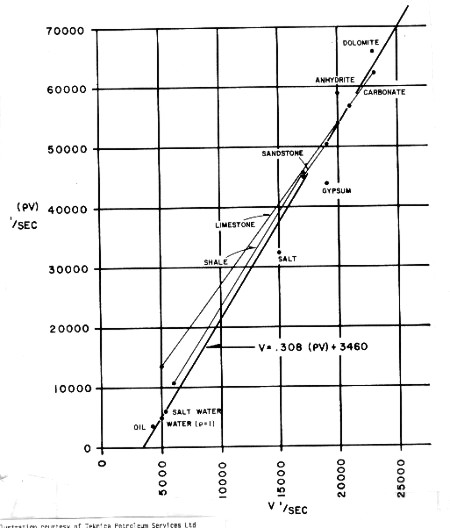Acoustic Impedance and Reflection Coefficients
Sound is reflected back toward the source of energy whenever an acoustic impedance boundary occurs or Poisson's ratio changes. Acoustic impedance is the product of velocity and density. Energy is also lost due to reflection and spherical divergence.

The SI unit of acoustic impedance is the pascal second per cubic metre (Pa·s/m3) or the rayl per square metre (rayl/m2), while that of specific acoustic impedance is the pascal second per metre (Pa·s/m) or the rayl.

The basic equation is:
0: Zp = Velocity * Density

Where:
Zp = specific acoustic impedance (rayls)
Velocity of sound in the material (m/s)
Density = density of the material (kg/m3)

In terms of well log measurements, for near vertical incidence :
1: Zp1 = KD4 * DENS1 / (DTC1 * KS3)
2: Zp2 = KD4 * DENS2 / (DTC2 * KS3)
3: Refl = (Zp2 - Zp1) / (Zp2 + Zp1)
4: Atten = Prod (1 - Refl ^ 2)

Where:
KD4 = 1000 for Metric units     (DENS in kg/m3, DTC in usec/m)
KD4 = 10^6 for English units   (DENS in g/cc, DTC in usec/ft)
KS3 = 1.00 for Metric units
KS3 = 3.281 for English units

For non-vertical incidence:
5: K = (Vavg - Vo) / DEPTH
6: ANGLE = Arctan ((DEPTH * X + Vo * X / K) / (DEPTH^2 + 2 * Vo * DEPTH / K - X^2 / 4))
7: Vrat = Vc2 / Vc1
OR 7A: Vrat = DTC1 / DTC2
8: Drat = DENS2 / DENS1
9: C = (Vrat^2 + (1 - Vrat^2) / (Cos(ANGLE))^2) ^ 0.5
10: Refl = (1 - Vrat * Drat * C) / (1 + Vrat * Drat * C)

The reflection coefficient will vary with incidence angle, equivalent to a variation with offset distance. Attenuation is seldom applied to reflection coefficient data, as synthetics are often compared to gain equalized data, in which attenuation has been compensated.

If density log data is missing or cannot be used due to bad hole conditions, an appropriate constant value or a value derived from the empirical chart below can be used. A complete reconstruction of the density log can be made if lithology is known, by using the modeling equations given in earlier Section.Empirical acoustic impedance from velocity

Page Views ---- Since 01 Jan 2015
Copyright 2023 by Accessible Petrophysics Ltd.
CPH Logo, "CPH", "CPH Gold Member", "CPH Platinum Member", "Crain's Rules", "Meta/Log", "Computer-Ready-Math", "Petro/Fusion Scripts" are Trademarks of the Author